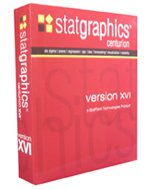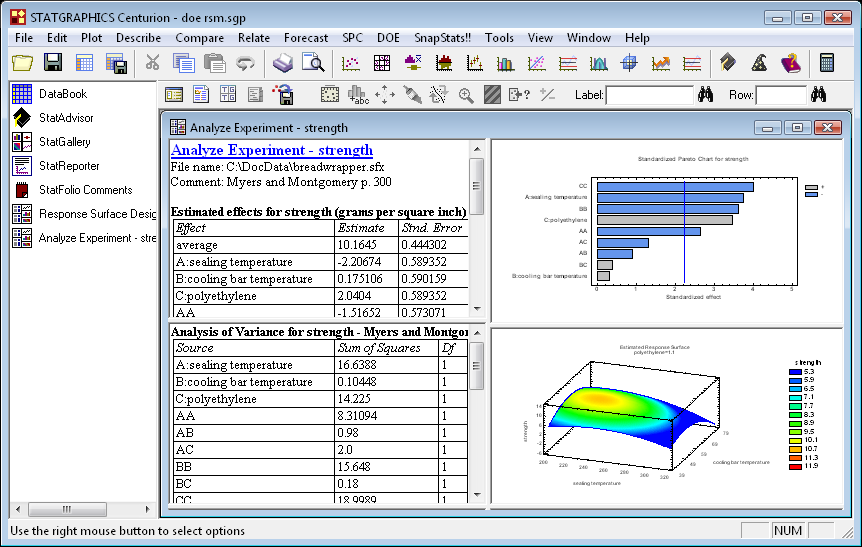# Statgraphics Centurion XVI

STATGRAPHICS Centurion XVI ContentsSTATGRAPHICS Centurion XVI is designed for anyone who wishes to do serious data analysis without investing weeks learning how to use a statistical package. It contains over 180 statistical procedures, covering everything from summary statistics to graphical representation of data. Yet you don't need to be a statistician to use the program. Everything is completely menu-driven, and there are tools such as the StatWizard and StatAdvisor to help you use the program most effectively.

STATGRAPHICS Centurion XVI is the 16th version of STATGRAPHICS for PC's. The first version was released in 1982. Our clients include many of the largest companies around the world looking to do graphical representation of data.

The statistical procedures in STATGRAPHICS Centurion XVI are divided into several major sections:

• Plot - Basic graphical representation of data plots, including scatterplots, matrix plots, exploratory plots, multivariate visualization techniques, time sequence plots, business charts, probability distribution plots, splines, 3D surface plots and contour plots.
• Describe - Descriptive statistics, including confidence intervals, hypothesis tests, tolerance limits, outlier identification, tests for normality, distribution fitting for censored and uncensored data, Weibull analysis and other life data analysis methods, tabulation and crosstabulation, multivariate methods such as PCA, factor analysis, cluster analysis, and correspondence analysis, nonparametric methods, time series smoothing and seasonal decomposition, and point process modeling for repairable systems.
• Compare - Comparison of more than one sample. Includes two sample comparisons for independent and paired samples, multiple sample comparison, ANOVA, variance components estimation, and a general linear models procedure.
• Relate - Regression and classification methods, including simple regression, polynomial regression, nonlinear regression, multiple and stepwise regression, calibration models, comparison of intercepts and slopes, ridge regression, PLS, logistic regression, probit analysis, Poisson and negative binomial regression, life data regression (parametic and nonparametric), discriminant analysis and a neural network Bayesian classifier.
• Forecast - Time series analysis and forecasting, including an automatic model selection procedure. Models include exponential smoothing, moving averages, random walks, linear and nonlinear trends, and ARIMA models.
• SPC - Methods for statistical process control, including Pareto charts, cause-and-effect diagrams, QFD, process mapping, capability analysis for variables and attributes, 27 types of control charts (including charts for multivariate data), gage R&R and linearity studies, and acceptance sampling.
• DOE - Design of experiments, including factorial and fractional factorial designs, Box-Behnken designs, central composite designs, Plackett-Burman designs, Draper-Lin designs, D-optimal designs, simple-lattice and simple-centroid designs for mixture experiments, robust parameter designs (Taguchi and Montgomery), BIB, randomized block, and Latin square designs for categorical factors. Analysis includes multi-response optimization and an interactive graphical response surface explorer.
• SnapStats - Streamlined versions of heavily used procedures such as Process Capability Analysis that produce a single preformatted page with six tables and graphs.
• Tools - Statistical tools including sample size determination, a Six Sigma calculator, sampling distributions, and Monte Carlo simulation.Editions
32-bit and 64-bit editions English, French, German, Italian, Spanish, Bilingual, Multilingual
List of statistics calculated and tests performed
User Manual in PDF format
Product brochure